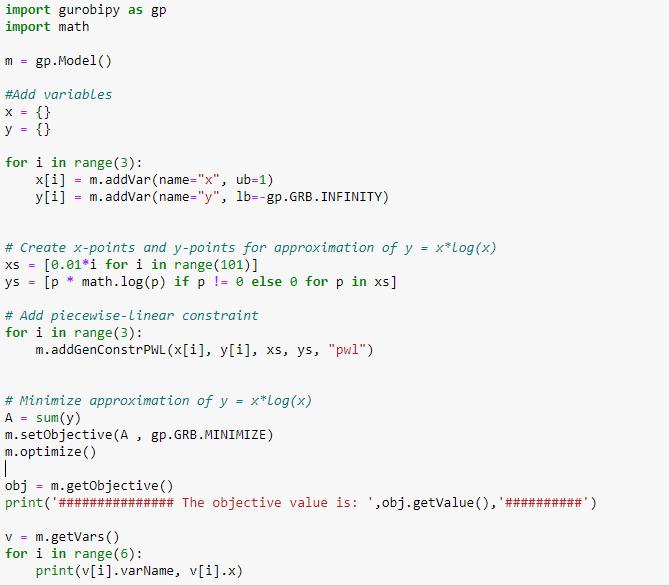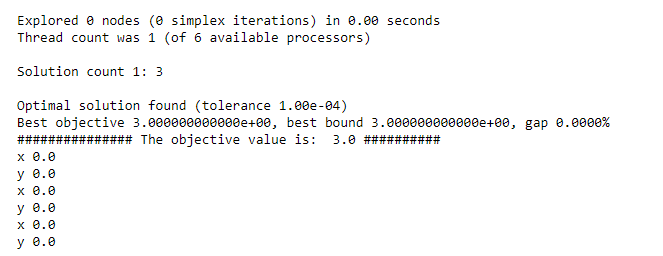•Gurobi Staff

Hi Yauhen,

Gurobi models general constraints like the logarithmic function using a piecewise-linear approximation of the function. Similarly, you could define your own piecewise-linear approximation of the function $$y = x \log(x)$$.

In the Python API, this can be done with the Model.addGenConstrPWL() method. Here is a (trivially solvable) example of minimizing a piecewise-linear approximation of $$y = x \log(x)$$ over the interval $$[0, 1]$$ (assuming $$x \log(x)$$ evaluates to $$0$$ at $$x=0$$ ):

import gurobipy as gpimport mathm = gp.Model()x = m.addVar(name="x", ub=1)y = m.addVar(name="y", lb=-gp.GRB.INFINITY)# Create x-points and y-points for approximation of y = x*log(x)xs = [0.01*i for i in range(101)]ys = [p*math.log(p) if p != 0 else 0 for p in xs]# Add piecewise-linear constraintm.addGenConstrPWL(x, y, xs, ys, "pwl")# Minimize approximation of y = x*log(x)m.setObjective(y, gp.GRB.MINIMIZE)m.optimize()

Gurobi finds the solution $$-0.36787334$$ to our approximate problem, which is not far from the optimal solution of the true problem, $$-\frac{1}{e} \approx -0.36787944$$:

Optimal solution found (tolerance 1.00e-04)Best objective -3.678733411372e-01, best bound -3.678733411372e-01, gap 0.0000%

You may want to try out different approximations (i.e., the number of breakpoints) depending on how large your problem is and the degree of approximation you are willing to accept. I would expect that approximating the $$p_i \log(p_i)$$ terms like this would work better than approximating each $$\log(p_i)$$ term and introducing bilinear terms into the objective.

I hope this helps!

Eli

•Yep, this seems to be exactly what I need. Thanks, Eli!

/Y.

•Hello Elli,

I have a question regarding the minimization of a multivariate function :

Actually, I used the same code as you suggested but with the objective to minimize sum( x[i] log(x[i])) instead of minimizing  : xlog(x) :To my surprise, I don't think the results are optimal.I don't know what I am missing ? and I'd like to understand how gurobi solves problems where there's a sum? Are there cases where the resolution is done in a decentralized manner, I mean, term by term?

Thank you.

Regards,

Ibtissam

•Gurobi Staff

Hi Ibtissam,

The issue is with this line:

A = sum(y)

You defined y to be a standard Python dictionary, and taking the sum of this dictionary object doesn't accomplish what you want:

>>> sum(y)3

To resolve this, you can write the summation as

A = gp.quicksum(y[i] for i in range(3))

Alternatively, you could take advantage of Gurobi's tupledict class by creating the $$y$$ variables using the Model.addVars() method, then using tupledict.sum() to obtain an expression for the summation of all of the $$y$$ variables:

y = m.addVars(3, lb=-gp.GRB.INFINITY, name="y")# ...m.setObjective(y.sum(), gp.GRB.MINIMIZE)

Either way, we get the optimal solution:

Optimal solution found (tolerance 1.00e-04)Best objective -1.103620023412e+00, best bound -1.103620023412e+00, gap 0.0000%

I hope this helps!

Eli

•Hello Eli,

Thank you for your response ! Indeed it worked. I do have one quick question, though : Is there any difference between gp.quicksum() and quicksum() ?

Because I remember using quicksum(y[i] for i in range(3)) but it didn't work.

Best Regards,

Ibtissam

•Gurobi Staff

Hi Ibtissam,

This depends on how you import the gurobipy package. Specifically, if you use

import gurobipy as gp

then the function is used with gp.quicksum(). If you import gurobipy using

from gurobipy import *

then the quicksum() function is imported directly into the main module's namespace. Note that it is generally regarded as bad practice to import packages in this manner.

Thanks,

Eli

•Dear all,

Maybe it is a bit late to comment in this post, but I am facing the very same problem in Scala.
I am new in Gurobi, and after several days, I don't know how to face it. I would like to remark that it has more than ten thousands variable.

I will be happy and very grateful if someone could help me.

My code, trying to imitate the example above, is

val env: GRBEnv = new GRBEnv("test.log")val model: GRBModel = new GRBModel(env)val x: GRBVar = model.addVar(1.0, GRB.INFINITY, 1.0, GRB.CONTINUOUS, "x")val y: GRBVar = model.addVar(-1.0, GRB.INFINITY, 1.0, GRB.CONTINUOUS, "y")val obj: GRBLinExpr = new GRBLinExpr()obj.addTerm(1.0, x)model.setObjective(obj, GRB.MINIMIZE)def createVar(stPoint: Double): LazyList[Double] = LazyList.cons(stPoint, createVar(stPoint+1.0))val xs: LazyList[Double] = createVar(0.01).take(200)val ys: List[Double] = xs.view.map(el => el*log(el)).toListmodel.addGenConstrPWL(x, y, xs.toArray, ys.toArray, "pwl")model.optimize()

but the solution is 1.0, which is not optimal.

Thanks a lot,

Bests,
Alejandro

•Gurobi Staff

The original Python code showed how to approximately solve $$\min \{ x \log(x) : x \in [0, 1]\}$$. Let's compare this to what your Scala code does.

val x: GRBVar = model.addVar(1.0, GRB.INFINITY, 1.0, GRB.CONTINUOUS, "x")val y: GRBVar = model.addVar(-1.0, GRB.INFINITY, 1.0, GRB.CONTINUOUS, "y")

This creates a continuous variable $$x$$ with bounds $$[1, +\infty)$$ and objective coefficient $$1$$, and a continuous variable $$y$$ with bounds $$[-1, +\infty)$$ and objective coefficient $$1$$. So, the bounds on $$x$$ are not correct.

After adding the two variables, the objective function is $$x + y$$. You replace this objective function with just $$x$$:

val obj: GRBLinExpr = new GRBLinExpr()obj.addTerm(1.0, x)model.setObjective(obj, GRB.MINIMIZE)

To match the Python example, we want the objective function to be $$y$$ (because $$y \approx x \log(x)$$).

Lastly, your code creates a piecewise-linear approximation of $$x \log(x)$$ using the following $$(x,y)$$ points:

(1.01, 0.0100498341616998) (2.01, 1.403250791362678)(3.01, 3.31683963706996)   (4.01, 5.569052877687096)                                (5.01, 8.073293934634835)  (6.01, 10.77848273876817)(7.01, 13.65083728433595)  (8.01, 16.66633299625294)(9.01, 19.80699899529842)  (10.01, 23.05888177920456)(11.01, 26.41083149758782) (12.01, 29.85373302946363)(13.01, 33.37999498574263) (14.01, 36.98319675848831)(15.01, 40.65783685113701) (16.01, 44.39914856740805)(17.01, 48.20296192299617) (18.01, 52.06559813697343)...

Since the domain of $$x$$ is $$[0, 1]$$, we want the x-values of the points defining our piecewise-linear approximation to sufficiently cover the interval $$[0, 1]$$.

Altogether, we have to fix the objective function, fix the bounds of $$x$$, and build a reasonable piecewise-linear approximation of $$x \log(x)$$:

val env: GRBEnv = new GRBEnv("test.log")val model: GRBModel = new GRBModel(env)// x \in [0, 1] with objective coefficient 0val x: GRBVar = model.addVar(0.0, 1.0, 0.0, GRB.CONTINUOUS, "x")// y \in [-1, \infty) with objective coefficient 1val y: GRBVar = model.addVar(-1.0, GRB.INFINITY, 1.0, GRB.CONTINUOUS, "y")// Build PWL approximation using 100 points spaced evenly on [0, 1]def createVar(stPoint: Double): LazyList[Double] = LazyList.cons(stPoint, createVar(stPoint+0.01))val xs: LazyList[Double] = createVar(0.01).take(100)val ys: List[Double] = xs.view.map(el => el*log(el)).toListmodel.addGenConstrPWL(x, y, xs.toArray, ys.toArray, "pwl")model.optimize()

This gives us an optimal solution of $$-0.3678733411372 \approx -\frac{1}{e} = \min \{ x\log(x) : x \in [0, 1]\}$$:

Optimal solution found (tolerance 1.00e-04)Best objective -3.678733411372e-01, best bound -3.678733411372e-01, gap 0.0000%
•Thanks a lot for such a helpful and complete answer! I am very grateful for that.

Bests,

Alejandro Mus

•Hello Eli,

I have a question regarding the minimization of a multivariate (2D) function :

Actually, I used the same code as you suggested but with the objective to minimize sum( log2(1 + ( a * x[i, j] / b *(d[i] - d[j]) ) ) ) instead of minimizing : xlog(x).

My problem is, when I put approximation between x and y points, Gurobi solver put error, i.e., "gurobipy.GurobiError: Arguments xpts and ypts must have the same length".

My code snippet:

p = SP_Global.addVars(U, V, vtype=GRB.CONTINUOUS, name="U")r = SP_Global.addVars(U, V, vtype=GRB.CONTINUOUS, name="V")# create p and r points for approximation of r = log2(1+ap/b)p_x_pts = [0.01*i for i in range(201)]r_y_pts = [np.log2(1 + (pow * xi_0)/(sigma * (np.abs((x1[i] - x2[j]) ** 2 + (y1[i] - y2[j]) ** 2 + z1[i] ** 2))))             for pow in p_x_pts for i in range(U) for j in range(V)]for i in range(U):    for j in range(V):        SP_Global.addGenConstrPWL(p[i, j], r[i, j], p_x_pts, r_y_pts, "pwl")

•Gurobi Staff

In each call to Model.addGenConstrPWL(), you need to break up the list of y-points ($$\texttt{r_y_pts}$$) so its length matches that of the x-points list ($$\texttt{p_x_pts}$$). I see Jaromił provided you with some sample code in another community post.

•Thank you Eli Towle

The code is working now.